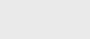1个月前 (10-23) |   抢沙发  22

[收起] 文章目录

## 什么是方法句柄？

1. 创建lookup
2. 创建method type
3. 查找方法句柄
4. 调用方法句柄

## 创建Lookup

``MethodHandles.Lookup publicLookup = MethodHandles.publicLookup();``

``````MethodHandles.Lookup lookup = MethodHandles.lookup();
``````

## 创建MethodType

MethodType的结构很简单，它由一个返回类型和适当数量的参数类型组成，这些参数类型必须在方法句柄及其所有调用方之间正确匹配。

``````MethodType mt = MethodType.methodType(List.class, Object[].class);
``````

``````MethodType mt = MethodType.methodType(int.class, Object.class);
``````

## 找到方法句柄

### 方法的MethodHandle

``````MethodType mt = MethodType.methodType(String.class, String.class);
MethodHandle concatMH = publicLookup.findVirtual(String.class, "concat", mt);``````

### 静态方法的方法句柄

``````MethodType mt = MethodType.methodType(List.class, Object[].class);

MethodHandle asListMH = publicLookup.findStatic(Arrays.class, "asList", mt);
``````

### 构造函数的方法句柄

``````MethodType mt = MethodType.methodType(void.class, String.class);

MethodHandle newIntegerMH = publicLookup.findConstructor(Integer.class, mt);``````

### 字段的方法句柄

``````public class Book {

String id;
String title;

// constructor

}``````

``````MethodHandle getTitleMH = lookup.findGetter(Book.class, "title", String.class);
``````

### 私有方法的方法句柄

`java.lang.reflect` API的帮助下，可以为私有方法创建方法句柄。

``````private String formatBook() {
return id + " > " + title;
}``````

``````Method formatBookMethod = Book.class.getDeclaredMethod("formatBook");
formatBookMethod.setAccessible(true);

MethodHandle formatBookMH = lookup.unreflect(formatBookMethod);``````

## 调用方法句柄

``````MethodType mt = MethodType.methodType(String.class, char.class, char.class);
MethodHandle replaceMH = publicLookup.findVirtual(String.class, "replace", mt);

String output = (String) replaceMH.invoke("jovo", Character.valueOf('o'), 'a');

assertEquals("java", output);``````

### 使用参数调用

``````MethodType mt = MethodType.methodType(List.class, Object[].class);
MethodHandle asList = publicLookup.findStatic(Arrays.class, "asList", mt);

List<Integer> list = (List<Integer>) asList.invokeWithArguments(1,2);

assertThat(Arrays.asList(1,2), is(list));
``````

### 调用Exact

``````MethodType mt = MethodType.methodType(int.class, int.class, int.class);
MethodHandle sumMH = lookup.findStatic(Integer.class, "sum", mt);

int sum = (int) sumMH.invokeExact(1, 11);

assertEquals(12, sum);``````

## 使用数组

MethodHandles不仅用于字段或对象，还用于数组。事实上，使用`asSpreader（）`API，可以生成一个数组扩展方法句柄。

``````MethodType mt = MethodType.methodType(boolean.class, Object.class);
MethodHandle equals = publicLookup.findVirtual(String.class, "equals", mt);

assertTrue((boolean) methodHandle.invoke(new Object[] { "java", "java" }));``````

## 增强方法句柄

``````MethodType mt = MethodType.methodType(String.class, String.class);
MethodHandle concatMH = publicLookup.findVirtual(String.class, "concat", mt);

MethodHandle bindedConcatMH = concatMH.bindTo("Hello ");

assertEquals("Hello World!", bindedConcatMH.invoke("World!"));``````

## Java 9增强功能

• 查找函数–允许从不同上下文中查找类，并支持接口中的非抽象方法
• 参数处理——改进参数折叠、参数收集和参数传播功能
• 附加组合–添加循环（`loop``whileLoop``doWhileLoop`…），并通过`tryFinally`提供更好的异常处理支持

• 增加JVM编译器优化
• 实例化减少
• 在使用MethodHandles API时启用了精度

## 结论### 注册